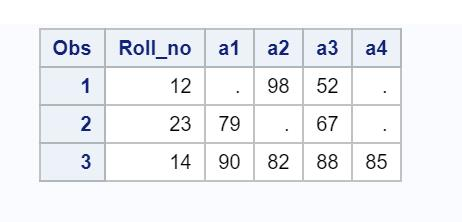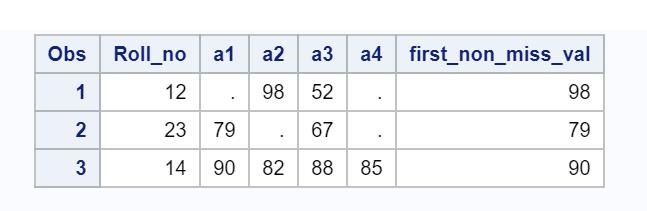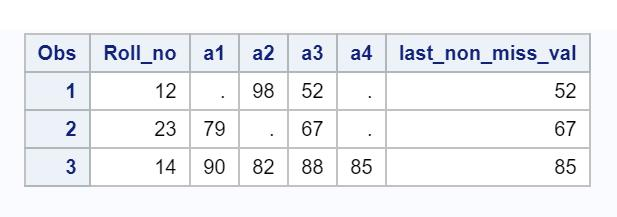# SAS | COALESCE Function with Examples

• Last Updated : 30 Jul, 2019

The COALESCE function is employed to pick the first non-missing value in a list of variables. In other words, it returns the first non-blank value of each row.

Let’s produce a sample dataset in SAS to know how COALESCE perform.

Example:

 `data ``temp``;``    ``input roll_no a1-a4;``    ``cards;12 . 98 52 . ``        ``23 79 . 67 . ``        ``14 90 82 88 85 ``;run;`

Output:• COALESCE : First Non-Missing Value

 `data exam;``set` `temp``;``first_non_miss_val = ``coalesce``(``of` `a1 - a4);``run;`

If you examine the output shown within the image below, you’d realize COALESCE returns 98 in first observation which is the first non-missing value among a1 = ., a2 = 98, a3 = 52, a4 =.• COALESCE : Last Non-Missing Value

Let us suppose you want to find out last non-missing value instead of first. For that, there is no such function available which will return last non-missing value but to achieve that we can reverse the list of variables and calculate the first non-missing value which would be equivalent to last non-missing value. Indirectly, we are considering to evaluate variables from right to left instead of left to right.

 `data exam;``set` `temp``;``last_non_miss_val = ``coalesce``(``of` `a4-a1);``run;`

Output:Note: coalesce(of a4-a1) is equivalent to coalesce(a4, a3, a2, a1).

My Personal Notes arrow_drop_up